# Simplifying a Formula with Ranges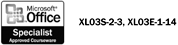You can simplify formulas by using ranges and range names . For example, if 12 cells on your worksheet contain monthly budget amounts, and you want to multiply each amount by 10%, you can insert one range address in a formula instead of inserting 12 different cell addresses, or you can insert a range name. Using a range name in a formula helps to identify what the formula does; the formula =TotalOrder*0.10, for example, is more meaningful than =SUM(F6:F19)*0.10.

#### Use a Range in a Formula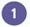Put your cursor where you would like the formula. Type an equal sign =SUM(.Click the first cell of the range, and then drag to select the last cell in the range. Excel enters the range address for you.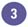Complete the formula by entering a close parentheses, or another function, and then click the Enter button.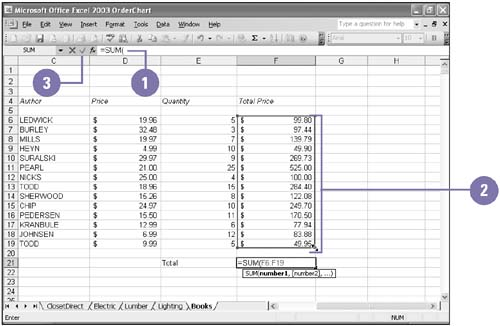#### Use a Range Name in a FormulaPut your cursor where you would like the formula. Type an equal sign =SUM(.Press F3 to display a list of named ranges.Click the name of the range you want to insert.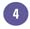Click OK.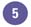Complete the formula by entering a close parentheses, or another function, and then click the Enter button.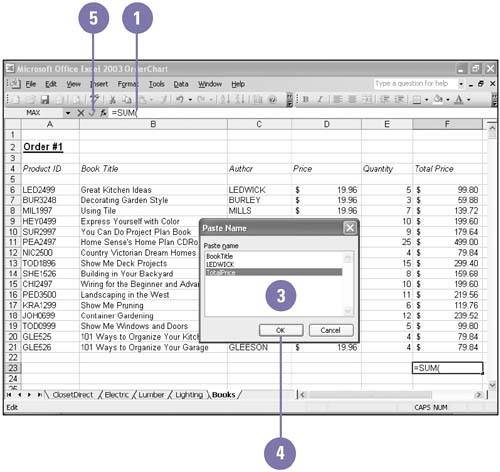Show Me Microsoft Office Excel 2003
ISBN: 0789730057
EAN: 2147483647
Year: 2002
Pages: 291